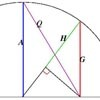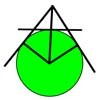# Resources tagged with: Arithmetic, Geometric and Harmonic Means

Filter by: Content type:
Age range:
Challenge level:

### There are 7 results

Broad Topics > Algebraic expressions, equations and formulae > Arithmetic, Geometric and Harmonic Means### Without Calculus

##### Age 16 to 18 Challenge Level:

Given that u>0 and v>0 find the smallest possible value of 1/u + 1/v given that u + v = 5 by different methods.### Pythagorean Golden Means

##### Age 16 to 18 Challenge Level:

Show that the arithmetic mean, geometric mean and harmonic mean of a and b can be the lengths of the sides of a right-angles triangle if and only if a = bx^3, where x is the Golden Ratio.### Three Ways

##### Age 16 to 18 Challenge Level:

If x + y = -1 find the largest value of xy by coordinate geometry, by calculus and by algebra.### Classical Means

##### Age 16 to 18 Challenge Level:

Use the diagram to investigate the classical Pythagorean means.### AMGM

##### Age 14 to 16 Challenge Level:

Can you use the diagram to prove the AM-GM inequality?